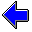Java Security Settings:
This web page employs Java, which requires specific security settings for correct operation.
If the applets on this page do not run correctly, consult the Virtual Chemistry Experiments FAQ
or the Physlet Physics web site for establishing the correct security settings.

# Phase Changes

## Phase Diagrams: Part 1

### Introduction

A phase diagram is common way to represent the various phases of a substance and the conditions under which each phase exists.

A phase diagram is a plot of pressure (P or ln P) vs temperature (T) in which lines on the diagram divide the plot into different regions. Each regions represents a particular phase and describes the conditions (T,P) under which this exists at equilibrium. Unless a point (T,P) lies on a line, only one phase may exist under these conditions at equilibrium.

The lines separating the regions describe conditions under which two phases may coexist at equilibrium; this situation constitutes a phase change. The line describing the solid-gas interface is given by

 ln P   =   - ΔHsubR T + ΔSsubR

in which ΔHsub and ΔSsub are the standard enthalpy and entropy of sublimation. A similar equation describes the line separating the liquid and gas regions.

A phase diagram is shown below. This particular substance has three phases: solid, liquid, and gas. Although a substance has only one liquid and one gas phase, it is possible to have multiple solid phases corresponding with different arrangements and orientations of molecules in the solid.

At the bottom of the diagram, a set of conditions (temperature and pressure) are shown. Locate this point on the phase diagram and determine the stable phase under these conditions. (Recall that pressing the left mouse button shows the position of the cursor on the graph.)

T/K:         P/atm:         ln P/atm:

 Phase Diagram Part 1 Part 2 Part 3 Part 4 Part 5Phase Changes Home PageVirtual Chemistry Home Page

PhaseDiagram1.html version 2.1
© 2001-2014 David N. Blauch